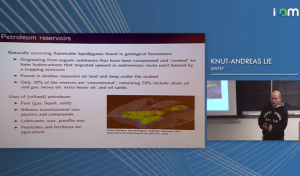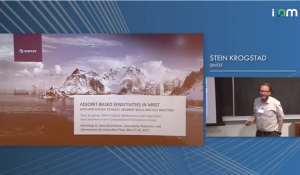﻿ Tutorial lectures

## MRST - MATLAB Reservoir Simulation Toolbox

Tutorial lectures
Here we have collected video recordings and slides for a few short courses and tutorials about MRST and reservoir simulation

### Knut-Andreas Lie: Introduction to reservoir simulation as practiced in industry, IPAM, UCLA, 2017This lecture discusses the three basic parts making up a reservoir simulation model: (i) a volumetric grid with cell/face properties that describes the given porous rock formation; (ii) a flow model given as a set of partial differential equations expressing conservation of mass or volumes together with appropriate closure relations; and (iii) a well model that describes the flow in and out of the reservoir.  In particular, we introduce the black-oil equations  and point out some numerical challenges involved in reservoir simulation.

### Stein Krogstad: Adjoint-based sensitivities in MRSTwith application to multi-segment well completion design, IPAM, UCLA, 2017The lecture explains how you can compute adjoint-based sensitivities in MRST. Sensitivities for reseroir controls have been around for a while, but here we also explain how to compute gradient and sensitivities with respect to parameters. We present a simple example of valve model scaling for a multisegment well and an analysis/matching of model parameters for the Sleipner CO2 injection.

### Multiscale Methods Summer School, Hasselt University, Belgium, June 2017

As part of a 4-day summer school on 'Upscaling techniques for mathematical models involving multiple scales' organised at Hasselt University, Knut-Andreas Lie gave four talks:

Published November 11, 2017